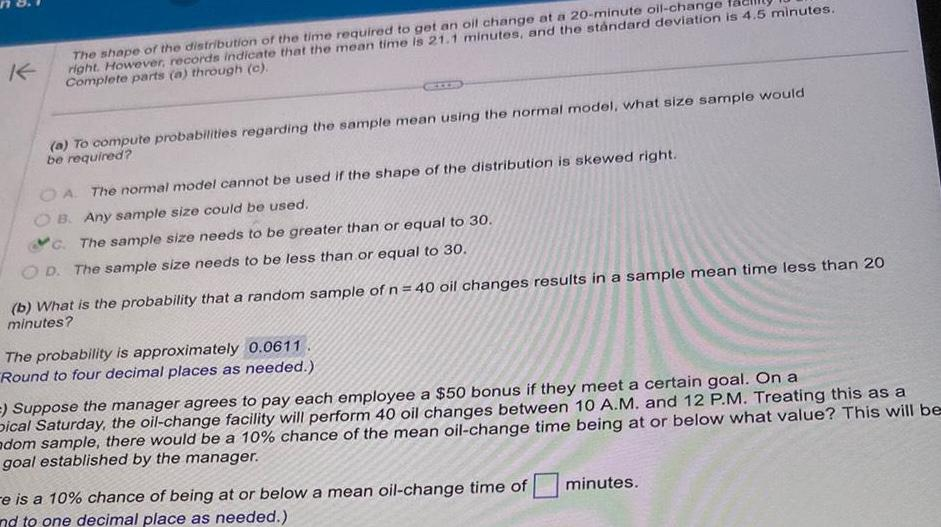Question:

# K The shape of the distribution of the time required to get

Last updated: 11/19/2023K The shape of the distribution of the time required to get an oil change at a 20 minute oil change right However records indicate that the mean time is 21 1 minutes and the standard deviation is 4 5 minutes Complete parts a through c a To compute probabilities regarding the sample mean using the normal model what size sample would be required OA The normal model cannot be used if the shape of the distribution is skewed right B Any sample size could be used C The sample size needs to be greater than or equal to 30 OD The sample size needs to be less than or equal to 30 b What is the probability that a random sample of n 40 oil changes results in a sample mean time less than 20 minutes The probability is approximately 0 0611 Round to four decimal places as needed Suppose the manager agrees to pay each employee a 50 bonus if they meet a certain goal On a Dical Saturday the oil change facility will perform 40 oil changes between 10 A M and 12 P M Treating this as a adom sample there would be a 10 chance of the mean oil change time being at or below what value This will be goal established by the manager e is a 10 chance of being at or below a mean oil change time of nd to one decimal place as needed minutes